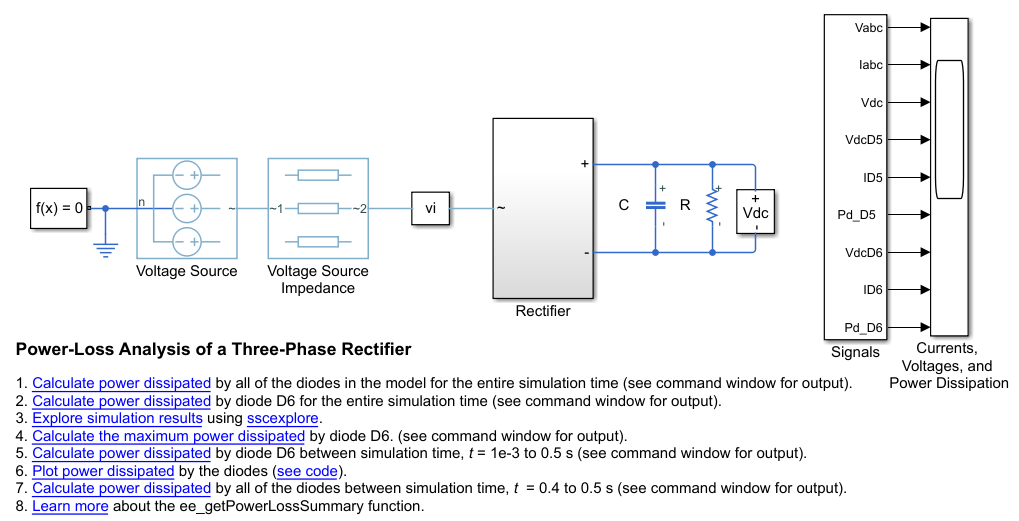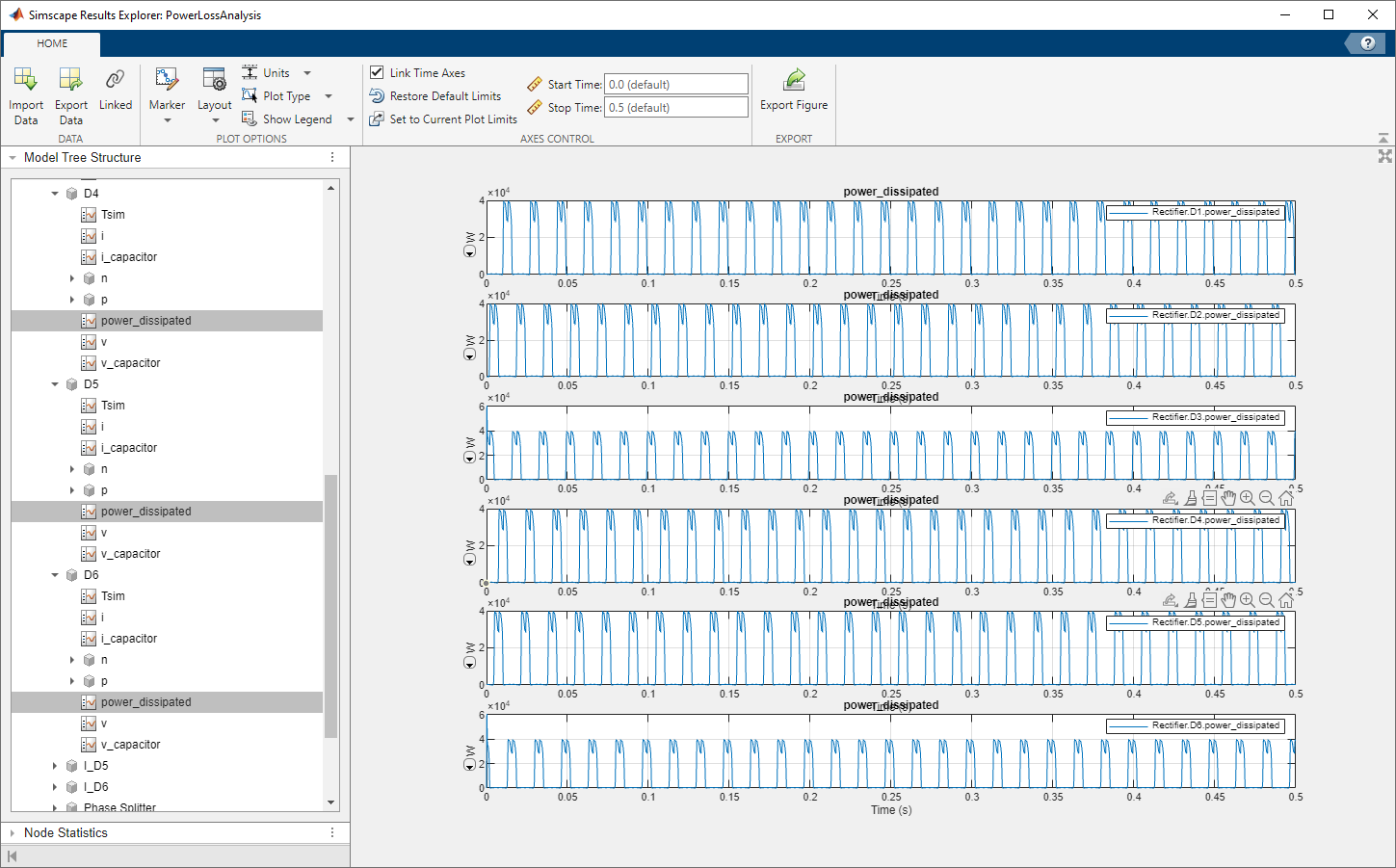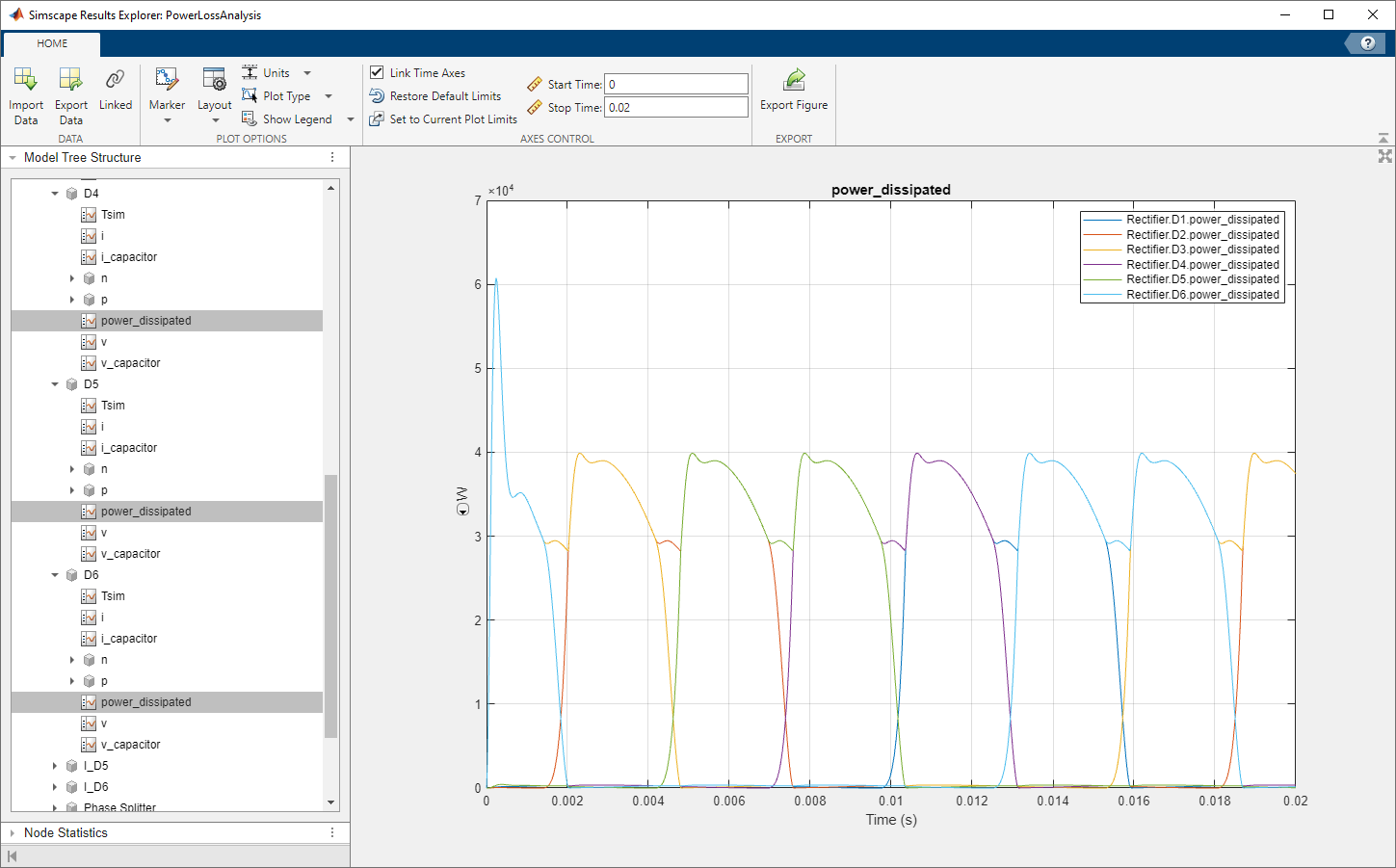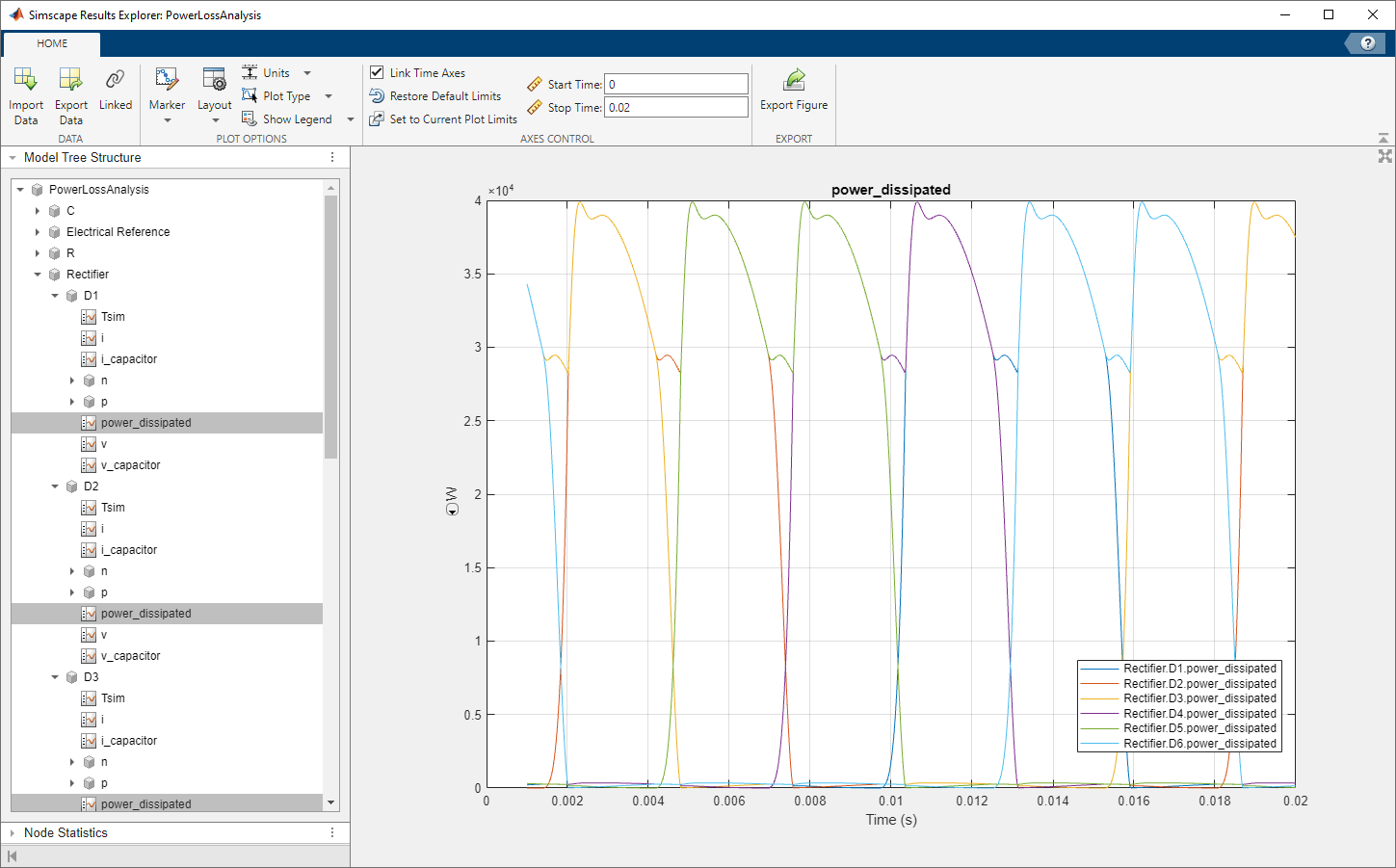## Perform a Power-Loss Analysis

This example shows how to analyze power loss and how to mitigate transient power dissipation behavior. Analyzing power loss, with and without transients, is useful for determining if components are operating within safety and efficiency guidelines.

### Prerequisite

This example requires a simulation log variable in your MATLAB® workspace. The model in this example is configured to log Simscape™ data for the whole model for the entire simulation time.

To learn how to determine if a model is configured to log simulation data, see Examine the Simulation Data Logging Configuration of a Model.

### Calculate Average Power Losses for the Simulation

1. Open the model. At the MATLAB command prompt, enter

`openExample("simscapeelectrical/PowerLossAnalysisExample");`2. Simulate the model.

`sim("PowerLossAnalysis")`

The simulation log variable, which is named `simlog_PowerLossAnalysis`, appears in the workspace.

3. Calculate the average losses for the entire simulation for each of the diodes in the model.

`rectifierLosses = ee_getPowerLossSummary(simlog_PowerLossAnalysis.Rectifier)`

```rectifierLosses = 6×2 table LoggingNode Power ________________ _____ {'Rectifier.D6'} 11514 {'Rectifier.D3'} 11514 {'Rectifier.D2'} 11511 {'Rectifier.D5'} 11511 {'Rectifier.D1'} 11511 {'Rectifier.D4'} 11511```

On average, diodes D3 and D6 dissipate more power than the other diodes in the rectifier.

### Analyze Power Dissipation Differences Using Instantaneous Power Dissipation

The Diode blocks each have a power_dissipated variable, which measures instantaneous power dissipation. To investigate the differences in the average power dissipated by the diodes, view the simulation data using the Simscape Results Explorer.

1. Open the simulation data using the Results Explorer.

`sscexplore(simlog_PowerLossAnalysis)`

2. View the instantaneous power dissipated by the diodes.

1. Expand the Rectifier node

2. Expand the D1 through D6 nodes

3. Click the `power_dissipated` nodes for diode D1, and then Ctrl+click the `power_dissipated` nodes for the other five diodes.

4. In the Results Explorer window, in the Plot Options section, click the Layout button and set it to `Separate`.At the beginning of the simulation, there is a difference in the power dissipation for each diode.

3. Take a closer look at the differences. Overlay the plots and zoom to the beginning of the simulation. In the Results Explorer window:

1. For Stop time, specify `0.02`.

2. Set Layout to `Overlay`.The variation in power dissipation is due to transient behavior at the beginning of the simulation. The model reaches steady state at simulation time, t ⋍ 0.001 seconds.

4. Determine the average power dissipation for only the diodes during the interval that contains transient behavior.

`rectifierLosses = ee_getPowerLossSummary(simlog_PowerLossAnalysis.Rectifier,0,1e-3)`
```rectifierLosses = 6×2 table LoggingNode Power ________________ ______ {'Rectifier.D3'} 38772 {'Rectifier.D6'} 38772 {'Rectifier.D4'} 293.62 {'Rectifier.D5'} 293.62 {'Rectifier.D1'} 139.11 {'Rectifier.D2'} 31.26```

The average power dissipated by diodes D3 and D6 exceeds the average for the other diodes.

5. Output a table of the maximum power dissipation for each diode, for the entire simulation time.

```pd_D1_max = max(simlog_PowerLossAnalysis.Rectifier.D1.power_dissipated.series.values); pd_D2_max = max(simlog_PowerLossAnalysis.Rectifier.D2.power_dissipated.series.values); pd_D3_max = max(simlog_PowerLossAnalysis.Rectifier.D3.power_dissipated.series.values); pd_D4_max = max(simlog_PowerLossAnalysis.Rectifier.D4.power_dissipated.series.values); pd_D5_max = max(simlog_PowerLossAnalysis.Rectifier.D5.power_dissipated.series.values); pd_D6_max = max(simlog_PowerLossAnalysis.Rectifier.D6.power_dissipated.series.values); diodes = {'D1';'D2';'D3';'D4';'D5';'D6'}; PowerMax = [pd_D1_max;pd_D2_max;pd_D3_max;pd_D4_max;pd_D5_max;pd_D6_max]; T = table(PowerMax,'RowNames', diodes)```
```T = 6×1 table PowerMax ________ D1 39892 D2 39892 D3 60746 D4 39891 D5 39892 D6 60746 ```

The maximum instantaneous power dissipation for diodes D3 and D6 is almost double the maximum instantaneous power dissipation for the other diodes.

### Mitigate Transient Effects in Simulation Data

To mitigate the transient power dissipation at the beginning of the simulation, use the final simulation state to initialize a new simulation at steady-state conditions.

1. Configure the model to save the final state.

1. Open the model configuration parameters.

2. In the Solver pane, change the Stop time from `0.5` to 1e-3.

3. In the Data Import/Export pane, select these options:

• Final States

• Save final operating point

4. Click

2. Run the simulation.

The final state is saved as the variable xFinal in the MATLAB workspace.

3. Configure the model to initialize using xFinal, in the model configuration parameters.

1. In the Data Import/Export pane:

• Select the Initial state option.

• Change the Initial state parameter value from `xInitial` to `xFinal`.

• Clear the Final states option.

2. In the Solver pane, change the Stop time to `0.5`.

3. Click .

4. Run the simulation.

5. View the data from the new simulation.

1. Click the button in the Simscape Results Explorer.

2. Click to confirm that `simlog_PowerLossAnalysis` is the variable name that contains the logged data.

3. To see the data more clearly, click and drag the legend away from the peak amplitudes.The plot shows that the simulation no longer contains the transient.

6. Output a table of the maximum power dissipation for each diode, for the modified simulation.

```pd_D1_max = max(simlog_PowerLossAnalysis.Rectifier.D1.power_dissipated.series.values); pd_D2_max = max(simlog_PowerLossAnalysis.Rectifier.D2.power_dissipated.series.values); pd_D3_max = max(simlog_PowerLossAnalysis.Rectifier.D3.power_dissipated.series.values); pd_D4_max = max(simlog_PowerLossAnalysis.Rectifier.D4.power_dissipated.series.values); pd_D5_max = max(simlog_PowerLossAnalysis.Rectifier.D5.power_dissipated.series.values); pd_D6_max = max(simlog_PowerLossAnalysis.Rectifier.D6.power_dissipated.series.values); diodes = {'D1';'D2';'D3';'D4';'D5';'D6'}; PowerMax = [pd_D1_max;pd_D2_max;pd_D3_max;pd_D4_max;pd_D5_max;pd_D6_max]; T = table(PowerMax,'RowNames', diodes)```
```T = 6×1 table PowerMax ________ D1 39892 D2 39892 D3 39891 D4 39891 D5 39892 D6 39892 ```

The maximum instantaneous power dissipation for diodes D3 and D6 is the same as the maximum instantaneous power dissipation for the other diodes.